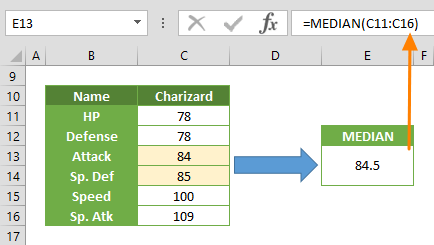The MEDIAN Excel function is a Math formula that calculates and returns the median of the selected values. The median in statistics represents the middle value in a dataset. In this guide, we’re going to show you how to use the Excel MEDIAN function and also go over some tips and error handling methods.

## Supported versions

• All Excel versions

MEDIAN(array)

## Arguments

 number1 The first number or numbers you want to calculate. You can enter a static value like 4, a cell reference like B6, or a cell range like B2:B8. [number2] Optional. The second number(s) you want to include in the calculation - up to 255 values.

## Examples

### Odd number of values

The Excel MEDIAN function only requires you to target the numbers or references in which you want to find the median. Static numbers, named ranges, individual cells, or range references can be used. In this example, we used the range reference C19:C23 which contains 5 numbers.

=MEDIAN(C19:C23)

The formula to find the median of a data set is {(n + 1) ÷ 2}th, where n is the number of values in the data set. Thus, the MEDIAN function will return (5 + 1) ÷ 2 = 3rd value as the smallest number in our range. The result is 84 in {78;78;84;85;100}.### Even number of values

If the number count in array is even, the mathematical median formula returns the number in between the two middle values. For example, the range C11:C16 contains 6 cells. As a result, the number should be (6 + 1) ÷ 2 = 3.5th for the smallest number in a range. This means that the median is the average of the 3rd and 4th numbers. The result is 84.5 for the data set {78;78;84;85;100;109}.

=MEDIAN(C11:C16)## Summary and Tips

• Median is not the average of values. Use the AVERAGE function to find the average value of your data.